2017年12月28日，中国一汽和中国扶贫开发协会精准扶贫战略合作签约仪式在北京隆重举行。

为促进教育平等，缩小贫富差距对青少年的影响，红旗品牌将在2018-2020年这三年中，对长征路沿线105个国家级贫困县的深度贫困学校做帮扶建设。
2017年12月28日，中国第一汽车集团有限公司（以下简称中国一汽）和中国扶贫开发协会在北京举行了精准扶贫战略合作框架协议签约仪式。中国第一汽车集团有限公司总经理助理况锦文、品牌公关部部长杜晓东，中国扶贫开发协会会长袁文先、副会长裴希更、法人兼副会长黄忠明，以及来自全国的媒体记者出席了本次活动。此次双方的精准扶贫战略合作框架协议签约及落地呈现，是中国一汽在9月21日发布“红旗扶贫梦想基金”后，在脱贫攻坚领域主动承担的又一次实质性行动。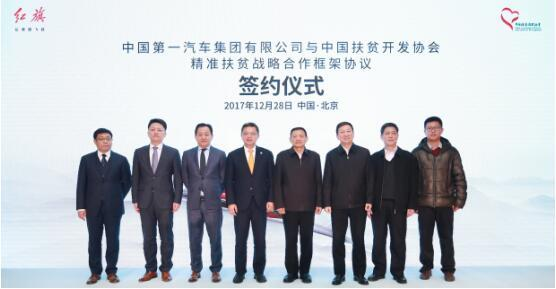结合“十九大” 走好新时代长征路
十九大召开过后，中国扶贫开发协会和中国一汽沿着党的宗旨，贯彻落实党在十九大中关于“坚决打赢脱贫攻坚战”的精神，拿出实际行动，共同承担贯穿新时代始终的这一份沉甸甸的责任。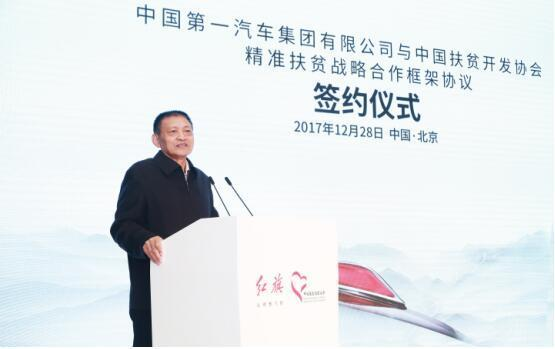本次签约仪式上，中国扶贫开发协会会长袁文先指出：“当前脱贫攻坚正处于‘攻坚拔寨’、‘啃硬骨头’的关键时期，当前脱贫形势依然严峻，全国范围内仍有接近4000万贫困人口，需要动员更多的爱心力量参与。中国扶贫开发协会与中国一汽在多次沟通和协商的基础上，就‘高举红旗，精准扶贫，走好新时代长征路’合作达成共识，这是具有历史意义和现实意义的战略举措，也是中国一汽勇于担当，履行央企政治责任和社会责任的真实写照。“
立足教育平等 建设和谐家园
在签约仪式上，中国第一汽车集团有限公司总经理助理况锦文深度阐述了红旗积极行动促进社会平等的责任与心愿，他表示：“作为中央企业，中国一汽不仅努力推动中国经济前行，促进中国汽车产业健康发展，还始终致力于履行央企的政治责任、经济责任和社会责任。”自2010年起中国一汽开始承担国家扶贫任务，截至目前已向对口帮扶的市、县、村派出挂职干部、村第一书记和专业技术人员50余人次，累计投入资金超过3.1亿元，实施精准扶贫项目90余个，直接和间接帮助超过10万人实现脱贫。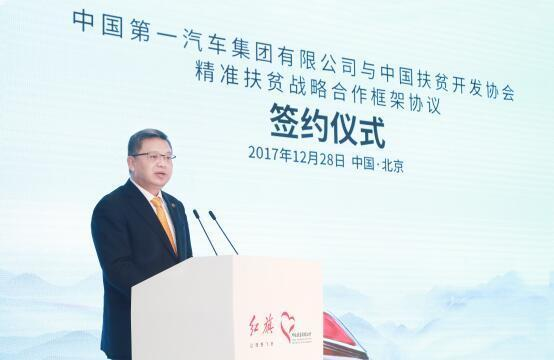中国一汽在多年来的公益行动中，不断融合和探索，“红旗扶贫梦想基金”的设立，将延伸扶贫公益的道路，通过打造社会扶贫平台，中国一汽号召更多人关注并参与到帮助深度贫困群体的行动中来，推动教育平等理念落地，从慈善公益1.0到倡导更多人参与的战略公益3.0，中国一汽正在让更多的贫困群体受益。
提升扶智扶贫 战略合作落定
双方正式签署了《中国第一汽车集团有限公司与中国扶贫开发协会精准扶贫战略合作框架协议》，宣布双方共同开展“高举红旗，精准扶贫，走好新时代长征路”项目。根据协议，中国一汽将在2018-2020这三年当中，在长征路沿线105个国家级贫困县的深度贫困县开展教育扶贫，从共享全社会优质教育资源、提升师资水平、捐建新型智能图书馆、打造梦想艺术课堂、帮扶特困高中生完成学业等方面发力，通过信息化教育体系建设，帮助他们获得平等的师资资源和发挥所长的舞台，提升贫困地区整体教育水平，帮助青少年开阔眼界，增强志气。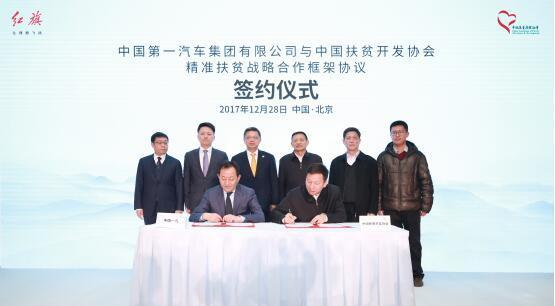据悉，目前项目落地的首家学校——江西省于都县车溪初中已开始接受该项目的改造。该学校初创于1957年，现有教职工68人，学生600人，住宿生400人，是于都县农村初中中等规模寄宿制学校。由于资金紧缺，办学条件存在重大欠缺：学生住宿条件较差，各功能教室未建立，配套严重落后，“红旗扶贫梦想基金”首期对音乐教室、智慧图书馆、体育活动室、美术教室及校园进行整体改造，为学校师生们提供更干净整洁的教学环境。
今天的签约仪式意味着“高举红旗，精准扶贫，走好新时代长征路”正式开启，红旗品牌将以人为本，着眼于未来，通过帮助贫困地区整体教育水平提升，为孩子们营造良好的教育环境，让孩子们用智慧武装头脑，用知识创造未来。面向未来，红旗品牌将秉持对国家、对社会、对环境、对未来负责任的坚定理念，与大家一起，让梦想成真，让理想飞扬。

##### 相关资讯

|分享

•已阅！握爪
•我手滑为你点赞
•128个赞！
•不明觉厉
•阅后既醉
•有钱！任性
•照片太美，我不敢看
•干货！杠杠的
•高大上
•膜拜中！
•请接受我的膝盖
•猫哥/猫妹么么哒
•神吐槽
•我只笑笑不说话
•我想静静
•窒息
##### 资讯相关车系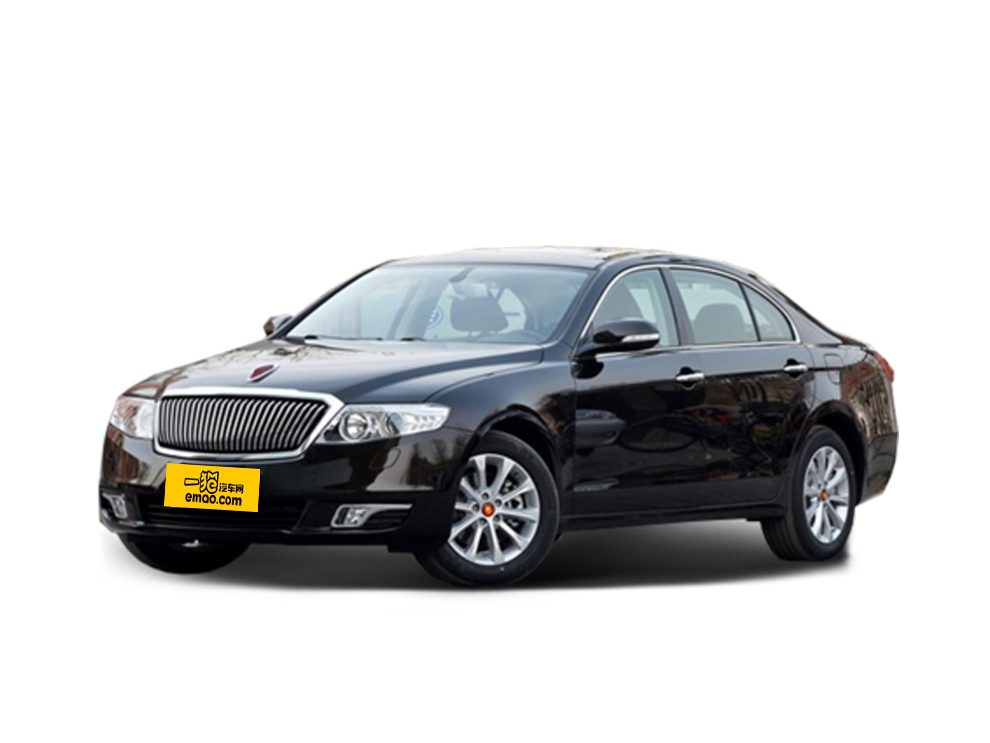##### 热度排行• 阿斯顿·马丁
• 奥迪
• 阿尔法罗密欧
• ALPINA

• 巴博斯
• 宝骏
• 宝马
• 保时捷
• 北汽制造
• 奔驰
• 奔腾
• 本田
• 比亚迪
• 标致
• 别克
• 宾利
• 布加迪
• 北汽威旺
• 北京
• 北汽绅宝
• 北汽幻速
• 北汽新能源
• 宝沃
• 比速汽车
• 北汽道达

• 昌河
• 长安
• 长城
• 长安商用
• 成功汽车
• 长江EV
• 长安轻车型

• 大众
• 道奇
• 东风
• 东风风神
• 东风小康
• 东南
• DS
• 东风风行
• 东风风度
• 东风风光

• 法拉利
• 菲亚特
• 丰田
• 福迪
• 福特
• 福田汽车
• 福汽启腾
• 风诺

• GMC
• 广汽传祺
• 广汽吉奥
• 观致

• 哈飞
• 海格
• 海马
• 华泰
• 黄海
• 恒天
• 红旗
• 哈弗
• 华颂
• 华凯
• 华泰新能源
• 汉腾汽车

• Jeep
• 江淮
• 江铃
• 捷豹
• 金杯
• 九龙
• 吉利汽车
• 金旅
• 金龙
• 江铃集团轻汽
• 江铃集团新能源
• 君马汽车
• 捷途

• 开瑞
• 凯迪拉克
• 科尼赛克
• 克莱斯勒
• KTM
• 卡威
• 凯翼
• 康迪
• 康迪电动汽车集团

• 猎豹汽车
• 兰博基尼
• 劳斯莱斯
• 雷克萨斯
• 雷诺
• 理念
• 力帆
• 莲花汽车
• 林肯
• 铃木
• 陆风
• 路虎
• 路特斯
• 领志
• 领克
• 零跑汽车

• MG
• MINI
• 马自达
• 玛莎拉蒂
• 迈凯伦
• 摩根

• 纳智捷

• 讴歌
• 欧宝
• 欧朗
• 欧拉
• 欧尚汽车

• 帕加尼

• 奇瑞
• 启辰
• 起亚
• 前途
• 庆铃汽车

• 日产
• 荣威
• 瑞麒汽车
• 如虎
• 瑞驰

• 上汽大通
• smart
• 三菱
• 双环
• 双龙
• 斯巴鲁
• 斯柯达
• 萨博
• 思铭
• 赛麟
• SWM斯威汽车

• TESLA
• 泰卡特
• 腾势

• 威麟
• 威兹曼
• 沃尔沃
• 五菱汽车
• 五十铃
• 潍柴英致
• WEY
• 蔚来
• 威马汽车

• 现代
• 雪佛兰
• 雪铁龙
• 西雅特
• 新特汽车
• 小鹏汽车
• 新宝骏

• 野马汽车
• 一汽
• 依维柯
• 英菲尼迪
• 永源
• 驭胜

• 中华
• 中兴
• 众泰
• 知豆
• 之诺
• 正道汽车
• A
• B
• C
• D
• E
• F
• G
• H
• I
• J
• K
• L
• M
• N
• O
• P
• Q
• R
• S
• T
• U
• V
• W
• X
• Y
• Z

• 阿斯顿·马丁
• 奥迪
• 阿尔法罗密欧
• ALPINA

• 巴博斯
• 宝骏
• 宝马
• 保时捷
• 北汽制造
• 奔驰
• 奔腾
• 本田
• 比亚迪
• 标致
• 别克
• 宾利
• 布加迪
• 北汽威旺
• 北京
• 北汽绅宝
• 北汽幻速
• 北汽新能源
• 宝沃
• 比速汽车
• 北汽道达

• 昌河
• 长安
• 长城
• 长安商用
• 成功汽车
• 长江EV
• 长安轻车型

• 大众
• 道奇
• 东风
• 东风风神
• 东风小康
• 东南
• DS
• 东风风行
• 东风风度
• 东风风光

• 法拉利
• 菲亚特
• 丰田
• 福迪
• 福特
• 福田汽车
• 福汽启腾
• 风诺

• GMC
• 广汽传祺
• 广汽吉奥
• 观致

• 哈飞
• 海格
• 海马
• 华泰
• 黄海
• 恒天
• 红旗
• 哈弗
• 华颂
• 华凯
• 华泰新能源
• 汉腾汽车

• Jeep
• 江淮
• 江铃
• 捷豹
• 金杯
• 九龙
• 吉利汽车
• 金旅
• 金龙
• 江铃集团轻汽
• 江铃集团新能源
• 君马汽车
• 捷途

• 开瑞
• 凯迪拉克
• 科尼赛克
• 克莱斯勒
• KTM
• 卡威
• 凯翼
• 康迪
• 康迪电动汽车集团

• 猎豹汽车
• 兰博基尼
• 劳斯莱斯
• 雷克萨斯
• 雷诺
• 理念
• 力帆
• 莲花汽车
• 林肯
• 铃木
• 陆风
• 路虎
• 路特斯
• 领志
• 领克
• 零跑汽车

• MG
• MINI
• 马自达
• 玛莎拉蒂
• 迈凯伦
• 摩根

• 纳智捷

• 讴歌
• 欧宝
• 欧朗
• 欧拉
• 欧尚汽车

• 帕加尼

• 奇瑞
• 启辰
• 起亚
• 前途
• 庆铃汽车

• 日产
• 荣威
• 瑞麒汽车
• 如虎
• 瑞驰

• 上汽大通
• smart
• 三菱
• 双环
• 双龙
• 斯巴鲁
• 斯柯达
• 萨博
• 思铭
• 赛麟
• SWM斯威汽车

• TESLA
• 泰卡特
• 腾势

• 威麟
• 威兹曼
• 沃尔沃
• 五菱汽车
• 五十铃
• 潍柴英致
• WEY
• 蔚来
• 威马汽车

• 现代
• 雪佛兰
• 雪铁龙
• 西雅特
• 新特汽车
• 小鹏汽车
• 新宝骏

• 野马汽车
• 一汽
• 依维柯
• 英菲尼迪
• 永源
• 驭胜

• 中华
• 中兴
• 众泰
• 知豆
• 之诺
• 正道汽车
• A
• B
• C
• D
• E
• F
• G
• H
• I
• J
• K
• L
• M
• N
• O
• P
• Q
• R
• S
• T
• U
• V
• W
• X
• Y
• Z

• 阿斯顿·马丁
• 奥迪
• 阿尔法罗密欧
• ALPINA

• 巴博斯
• 宝骏
• 宝马
• 保时捷
• 北汽制造
• 奔驰
• 奔腾
• 本田
• 比亚迪
• 标致
• 别克
• 宾利
• 布加迪
• 北汽威旺
• 北京
• 北汽绅宝
• 北汽幻速
• 北汽新能源
• 宝沃
• 比速汽车
• 北汽道达

• 昌河
• 长安
• 长城
• 长安商用
• 成功汽车
• 长江EV
• 长安轻车型

• 大众
• 道奇
• 东风
• 东风风神
• 东风小康
• 东南
• DS
• 东风风行
• 东风风度
• 东风风光

• 法拉利
• 菲亚特
• 丰田
• 福迪
• 福特
• 福田汽车
• 福汽启腾
• 风诺

• GMC
• 广汽传祺
• 广汽吉奥
• 观致

• 哈飞
• 海格
• 海马
• 华泰
• 黄海
• 恒天
• 红旗
• 哈弗
• 华颂
• 华凯
• 华泰新能源
• 汉腾汽车

• Jeep
• 江淮
• 江铃
• 捷豹
• 金杯
• 九龙
• 吉利汽车
• 金旅
• 金龙
• 江铃集团轻汽
• 江铃集团新能源
• 君马汽车
• 捷途

• 开瑞
• 凯迪拉克
• 科尼赛克
• 克莱斯勒
• KTM
• 卡威
• 凯翼
• 康迪
• 康迪电动汽车集团

• 猎豹汽车
• 兰博基尼
• 劳斯莱斯
• 雷克萨斯
• 雷诺
• 理念
• 力帆
• 莲花汽车
• 林肯
• 铃木
• 陆风
• 路虎
• 路特斯
• 领志
• 领克
• 零跑汽车

• MG
• MINI
• 马自达
• 玛莎拉蒂
• 迈凯伦
• 摩根

• 纳智捷

• 讴歌
• 欧宝
• 欧朗
• 欧拉
• 欧尚汽车

• 帕加尼

• 奇瑞
• 启辰
• 起亚
• 前途
• 庆铃汽车

• 日产
• 荣威
• 瑞麒汽车
• 如虎
• 瑞驰

• 上汽大通
• smart
• 三菱
• 双环
• 双龙
• 斯巴鲁
• 斯柯达
• 萨博
• 思铭
• 赛麟
• SWM斯威汽车

• TESLA
• 泰卡特
• 腾势

• 威麟
• 威兹曼
• 沃尔沃
• 五菱汽车
• 五十铃
• 潍柴英致
• WEY
• 蔚来
• 威马汽车

• 现代
• 雪佛兰
• 雪铁龙
• 西雅特
• 新特汽车
• 小鹏汽车
• 新宝骏

• 野马汽车
• 一汽
• 依维柯
• 英菲尼迪
• 永源
• 驭胜

• 中华
• 中兴
• 众泰
• 知豆
• 之诺
• 正道汽车
• A
• B
• C
• D
• E
• F
• G
• H
• I
• J
• K
• L
• M
• N
• O
• P
• Q
• R
• S
• T
• U
• V
• W
• X
• Y
• Z